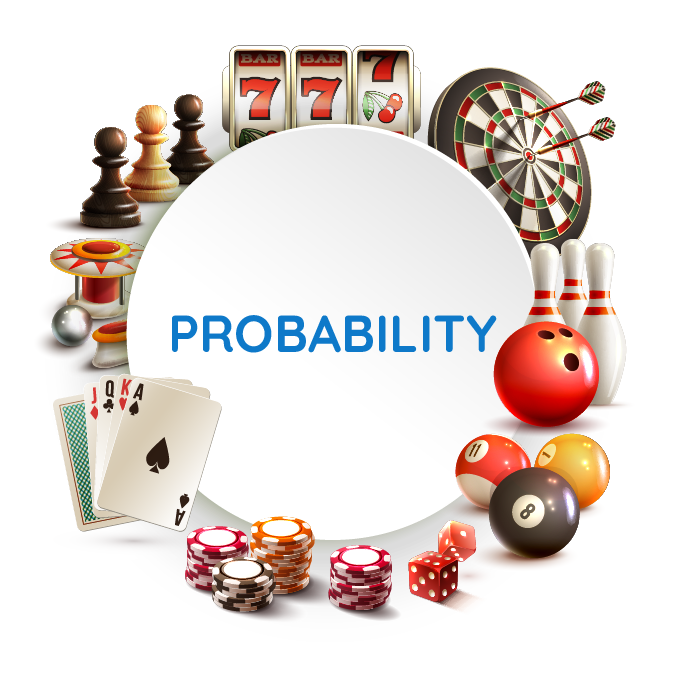There are three ways to assign probability. They are:

• Classical method
• Relative Frequency
• Subjective method

Optimization is seeking the best course of action. It is the act of making a decision that does not involve uncertainty.

WHY PROBABILITY

A decision can be made by:

• Optimization
• Sensitivity analysis
• With uncertainty

Data analysis can be in three formats namely:

• Descriptive
• Inferential
• Relationships

Uncertainty is resolved by measuring and modelling.

Managers often base their decisions on an analysis of uncertainties

What is the difference between uncertainty and risk?

In the case of uncertainty, you do not have all the information but in cases of risk, the information is available to you.

Probability is quantitative not qualitative. It can only be expressed from zero to one (0 – 1). A probability value cannot be negative.

The closer to 1, the probability of an event is, the more likely it is to occur.

The closer to 0, the probability of an event is, the less likely it is to occur. At 0.5, the occurrence of the event is just as likely as it is unlikely.

In statistics, the notion of an experiment differs somewhat from that of an experiment in the physical science.

Statistical experiments are sometimes called random experiments.

An experimental outcome is also called a sample point.

Sample space for an experiment is the set of all experimental outcome.

An experiment is any process that generates well defined outcomes.

The basic requirement for assigning probability

0P(Ei)1 for all

Where, Ei is the ith experimental outcome

P(Ei) is the probability

The probability assigned to each experimental outcome must be between 0 and 1, inclusively.

The probability of a sample space should sum up to 1.

The probability of all sample points should sum up to 1.

The different methods of probability

1. The classical method: this is the method of assigning probability based on the assumption of equally likely outcomes. For example, tossing a coin or throwing a dice.
2. Relative Frequency: this is method of assigning probability based on experimentation or historical data. The sum of events is divided by its frequency.
3. The subjective method: this is the method of assigning probability based on judgement. An event is a collection of sample points. The probability of any event is equal to the sum of probability of the sample points in the event.

Probability of events that are not part of a subspace is the complement of an event.

The probability of an event and its complement is equal to 1.

Exercise.

A mail order company tracks the number of returns it receives each day. The information for the last 50 days is shown below

1. How many sample points are there
2. List and assign probability to the sample points
3. What procedure was used to assign these probabilities

1. There are 4 sample points
2. The probabilities are 0.12, 0.4, 0.3 and 0.18 respectively for the number of returns. This was gotten by dividing the total number of days by the frequency. i.e 50/6 = 0.12  et.c
3. The procedure used to assign the probability is relative frequency method.

#MMBA3.## Understanding the Balance Sheet and the Income Statement: A Guide for Business Managers #MMBA5

in